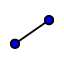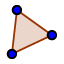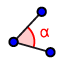# Rectangle Construction Template

## Use the tools below to construct a rectangle ABCD.

Construct both diagonals and of this rectangle and use the DISTANCE toolto measure their lengths. What do you notice?

## In the app above, construct the intersection of both diagonals. Label this point as E (like shown in this pic.)

Now right click on both diagonals and uncheck Show Object (to hide them). Then use the SEGMENT toolto construct segments , , , and . What do you notice?

Use the POLYGONtool to construct triangle AEB, CEB, DEC, and AED. Then use the ANGLEtool to measure the angles of each of these polygons. What do you notice?

In the picture (with graph paper background) above, which angles are congruent to angle 1?

Select all that apply
• A
• B
• C
• D
• E
• F
• G
• H
• I
• J
• K

In the picture (with graph paper background) above, which angles are congruent to angle 2?

Select all that apply
• A
• B
• C
• D
• E
• F
• G
• H
• I
• J
• K

What properties here hold true for ALL RECTANGLES?

Select all that apply
• A
• B
• C
• D
• E

What properties here hold true for RECTANGLES ONLY and are not necessarily true for all parallelograms?

Select all that apply
• A
• B
• C
• D
• E